# Mandalaxies

One cannot escape the feeling that these mathematical formulas have an independent existence and an intelligence of their own, that they are wiser than we are, wiser even than their discoverers (Heinrich Hertz)

I love spending my time doing mathematics: transforming formulas into drawings, experimenting with paradoxes, learning new techniques … and R is a perfect tool for doing it. Maths are for me a the best way of escape and evasion from reality. At least, doing maths is a stylish way of wasting my time.

When I read something interesting, many times I feel the desire to try it by myself. That’s what happened to me when I discovered this fabolous book by Julien C. Sprott. I cannot stop doing images with the formulas that contains. Today I present you a mix of mandalas and galaxies that I called Mandalaxies:

This time, the equation that drives these drawings is this one:$x_{n+1}= 10a_1+(x_n+a_2sin(a_3y_n+a_4))cos(\alpha)+y_nsin(\alpha)\\ y_{n+1}= 10a_5-(x_n+a_2sin(a_3y_n+a_4))sin(\alpha)+y_nsin(\alpha)$
where$\alpha=2\pi/(13+10a_6)$

The equation depends on six parameters (from a1 to a6). Searching randomly for values between -1.2 and 1.3 to each of them, you can generate an infinite number of beautiful images:

Here you can find the code to do your own images. Once again, Rcpp is key to generate the set of points to plot quickly since each of the previous plots contains 4 million points.

# Rcpp, Camarón de la Isla and the Beauty of Maths

Desde que te estoy queriendo
yo no sé lo que me pasa
cualquier vereda que tomo
siempre me lleva a tu casa
(Y mira que mira y mira, Camarón de la Isla)

The verses that head this post are taken from a song of Camarón de la Isla and illustrate very well what is a strange attractor in the real life. For non-Spanish speakers a translation is since I’m loving you, I don’t know what happens to me: any path I take, always ends at your house. If you don’t know who is Camarón de la Isla, hear his immense and immortal music.

I will not try to give here a formal definition of a strange attractor. Instead of doing it, I will try to describe them with my own words. A strange attractor can be defined with a system of equations (I don’t know if all strage attractors can be defined like this). These equations determine the trajectory of some initial point along a number of steps. The location of the point at step i, depends on the location of it at step i-1 so the trajectory is calculated sequentially. These are the equations that define the attractor of this experiment:$x_{n+1}= a_{1}+a_{2}x_{n}+a_{3}y_{n}+a_{4} |x_{n}|^{a_5}+a_{6} |y_{n}|^{a_7}\\ y_{n+1}= a_{8}+a_{9}x_{n}+a_{10}y_{n}+a_{11} |x_{n}|^{a_{12}}+a_{13} |y_{n}|^{a_{14}}$

As you can see there are two equations, describing the location of each coordinate of the point (therefore it is located in a two dimensional space). These equations are impossible to resolve. In other words, you cannot know where will be the point after some iterations directly from its initial location. The adjective attractor comes from the fact of the trajectory of the point tends to be the same independently of its initial location.

Here you have more examples: folds, waterfalls, sand, smoke … images are really appealing:

The code of this experiment is here. You will find there a definition of parameters that produce a nice example image. Some comments:

• Each point depends on the previous one, so iteration is mandatory; since each plot involves 10 million points, a very good option to do it efficiently is to use Rcpp, which allows you to iterate directly in C++.
• Some points are quite isolated and far from the crowd of points. This is why I locate some breakpoints with quantile to remove tails. If not, the plot may be reduced to a big point.
• The key to obtain a nice plot if to find out a good set of parameters (a1 to a14). I have my own method, wich involves the following steps: generate a random value for each between -4 and 4, simulate a mini attractor of only 2000 points and keep it if it doesn’t diverge (i.e. points don’t go to infinite), if x and y are not correlated at all and its kurtosis is bigger than a certain thresold. If the mini attractor overcome these filters, I keep its parameters and generate the big version with 10 million points.
• I would have publish this method together with the code but I didn’t. Why? Because this may bring yourself to develop your own since mine one is not ideal. If you are interested in mine, let me know and I will give you more details. If you develop a good method by yourself and don’t mind to share it with me, let me know as well, please.

This post is inspired in this beautiful book from Julien Clinton Sprott. I would love to see your images.

# Spinning Pins

Condenado a estar toda la vida, preparando alguna despedida (Desarraigo, Extremoduro)

I live just a few minutes from the Spanish National Museum of Science and Technology (MUNCYT), where I use to go from time to time with my family. The museum is plenty of interesting artifacts, from a portrait of Albert Einstein made with thousands of small dices to a wind machine to experiment with the Bernoulli’s effect. There is also a device which creates waves. It is formed by a number of sticks, arranged vertically, each of one ending with a ball (so it forms a sort of pin). When you push the start button, all the pins start to move describing circles. Since each pair of consecutive pins are separated by the same angle, the resulting movement imitates a wave. In this experiment I created some other machines. The first one imitates exactly the one of the museum:

If you look carefully only to one pin, you will see how it describes a circle. Each one starts at a different angle and all them move at the same speed. Although an individual pin is pretty boring, all together create a nice pattern. The museum’s machine is formed just by one row of 20 pins. I created a 20×20 grid of pins to make result more appealing.

Playing with the angle between pins you can create another nice patterns like these:

The code is incredibly simple and can be used as a starting point to create much more complicated patterns, changing the speed depending on time or the location of pins. Play with colors or shapes of points, the number of pins or with the separation and speed of them. The magic of movement is created with gganimate package. You can find the code here.

Merry Christmas and Happy New Year. Thanks a lot for reading my posts.

# Flowers for Julia

No hables de futuro, es una ilusión cuando el Rock & Roll conquistó mi corazón (El Rompeolas, Loquillo y los Trogloditas)

In this post I create flowers inspired in the Julia Sets, a family of fractal sets obtained from complex numbers, after being iterated by a holomorphic function. Despite of the ugly previous definition, the mechanism to create them is quite simple:

• Take a grid of complex numbers between -2 and 2 (both, real and imaginary parts).
• Take a function of the form$f(z)=z^{n}+c$ setting parameters$n$ and$c$.
• Iterate the function over the complex numbers several times. In other words: apply the function on each complex. Apply it again on the output and repeat this process a number of times.
• Calculate the modulus of the resulting number.
• Represent the initial complex number in a scatter plot where x-axis correspond to the real part and y-axis to the imaginary one. Color the point depending on the modulus of the resulting number after applying the function$f(z)$ iteratively.

This image corresponds to a grid of 9 million points and 7 iterations of the function$f(z)=z^{5}+0.364716021116823$: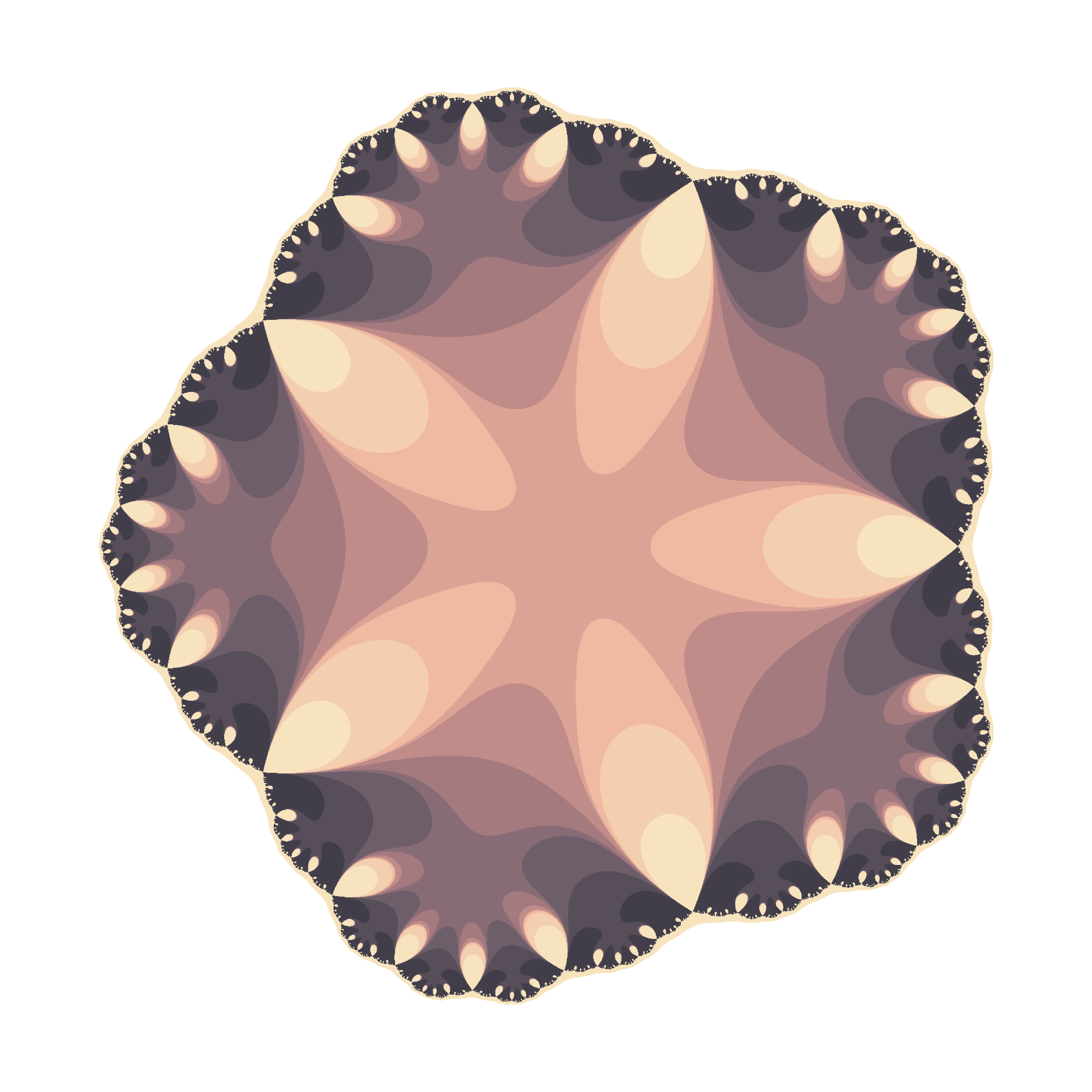To color the points, I pick a random palette from the top list of COLOURLovers site using the colourlovers package. Since each flower involves a huge amount of calculations, I use Reduce to make this process efficiently. More examples: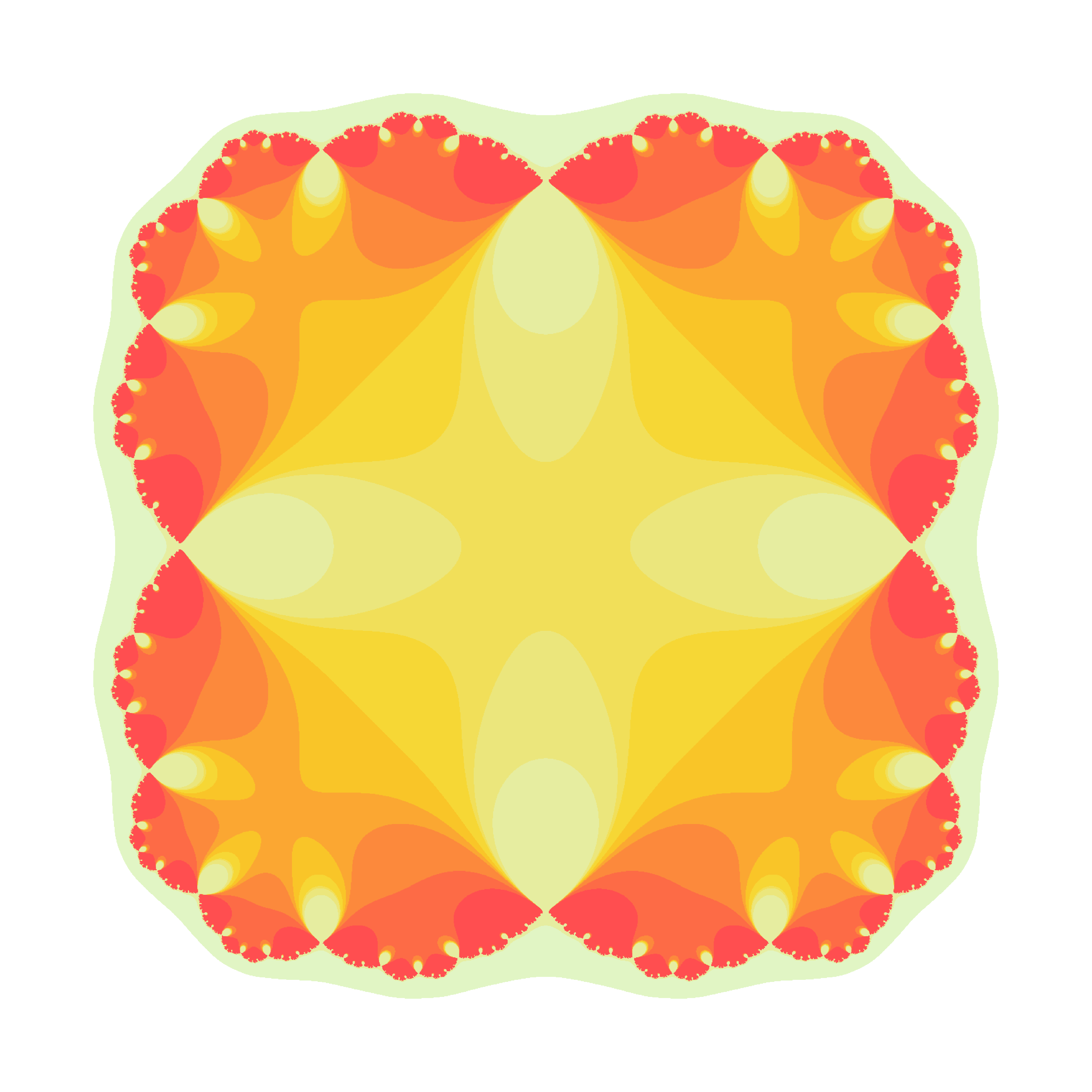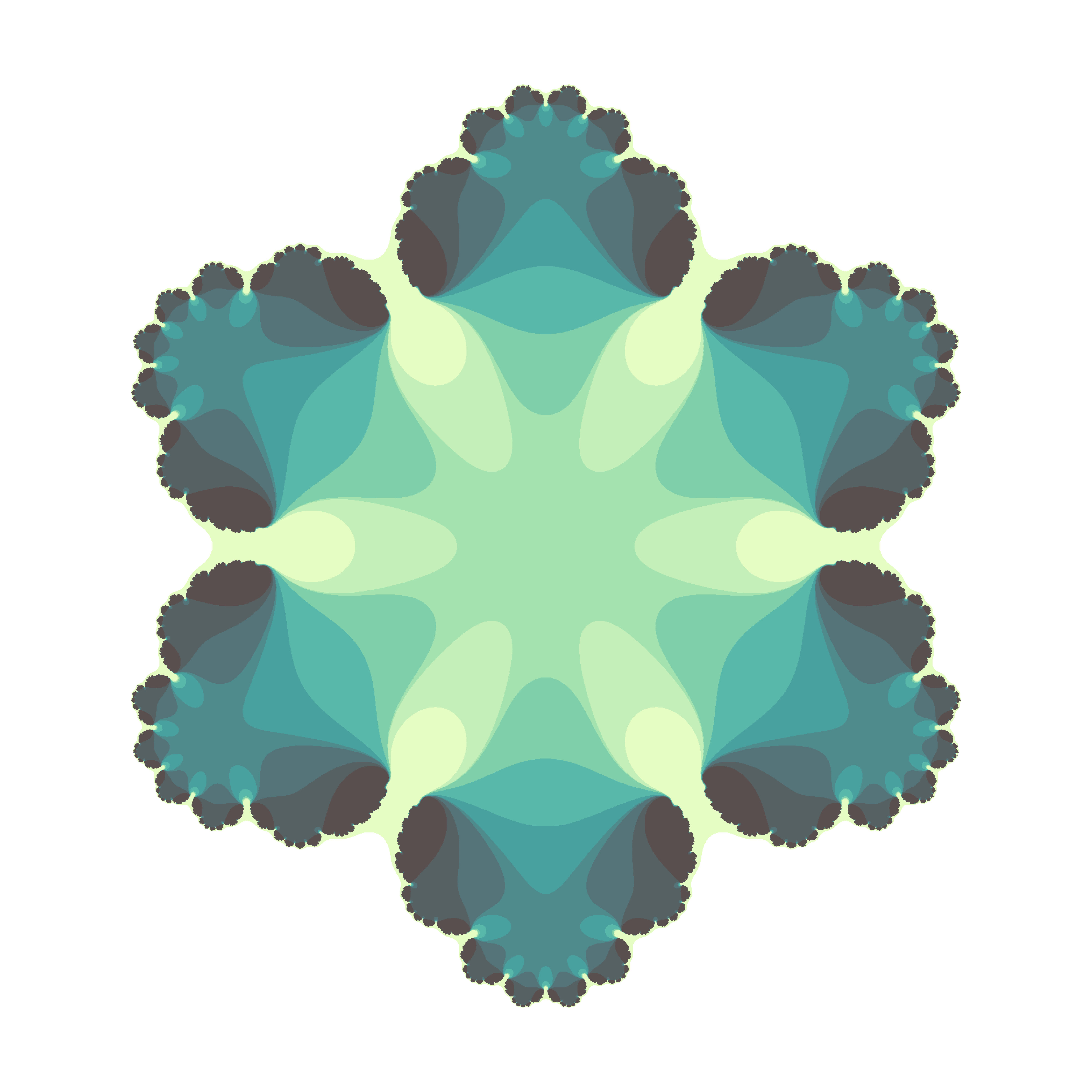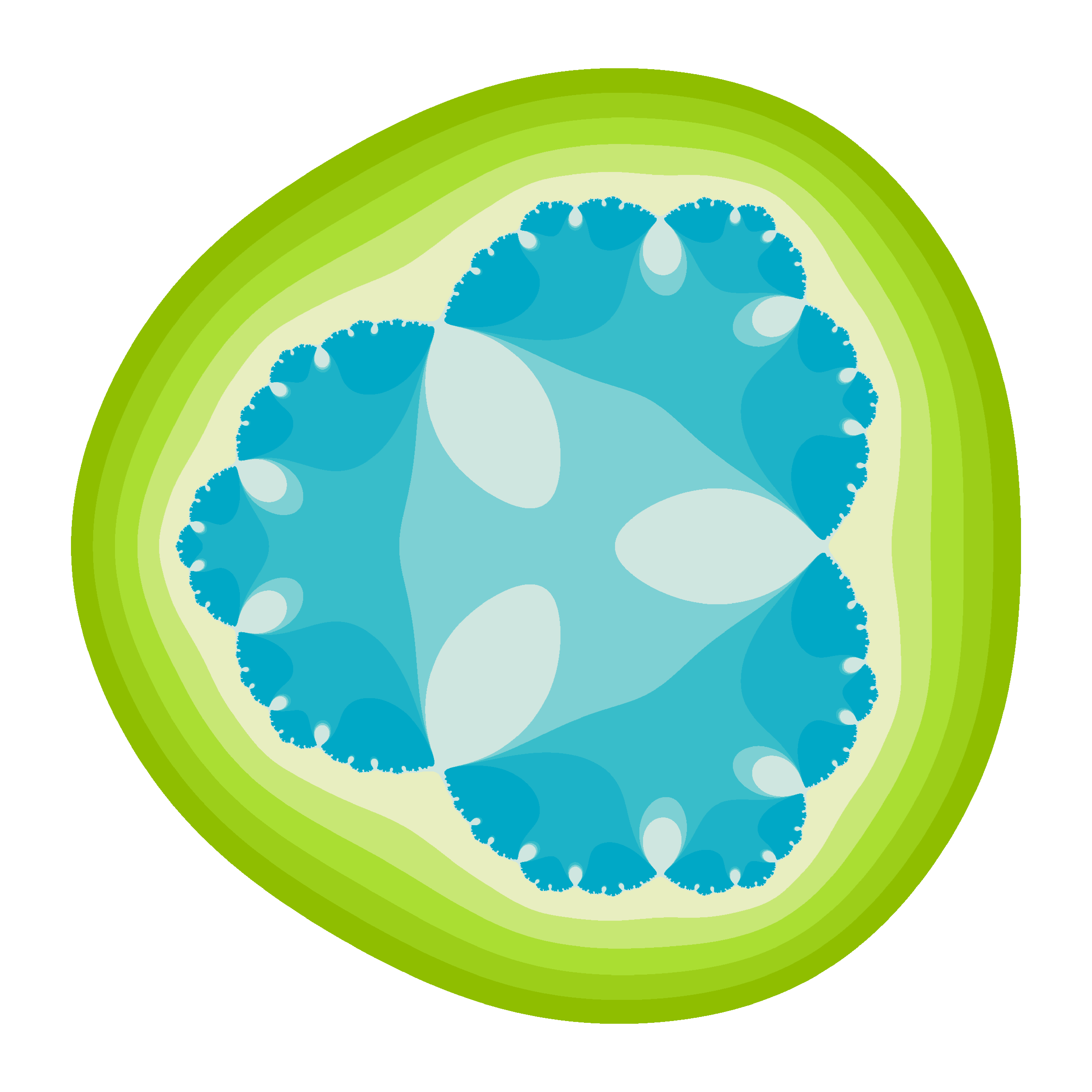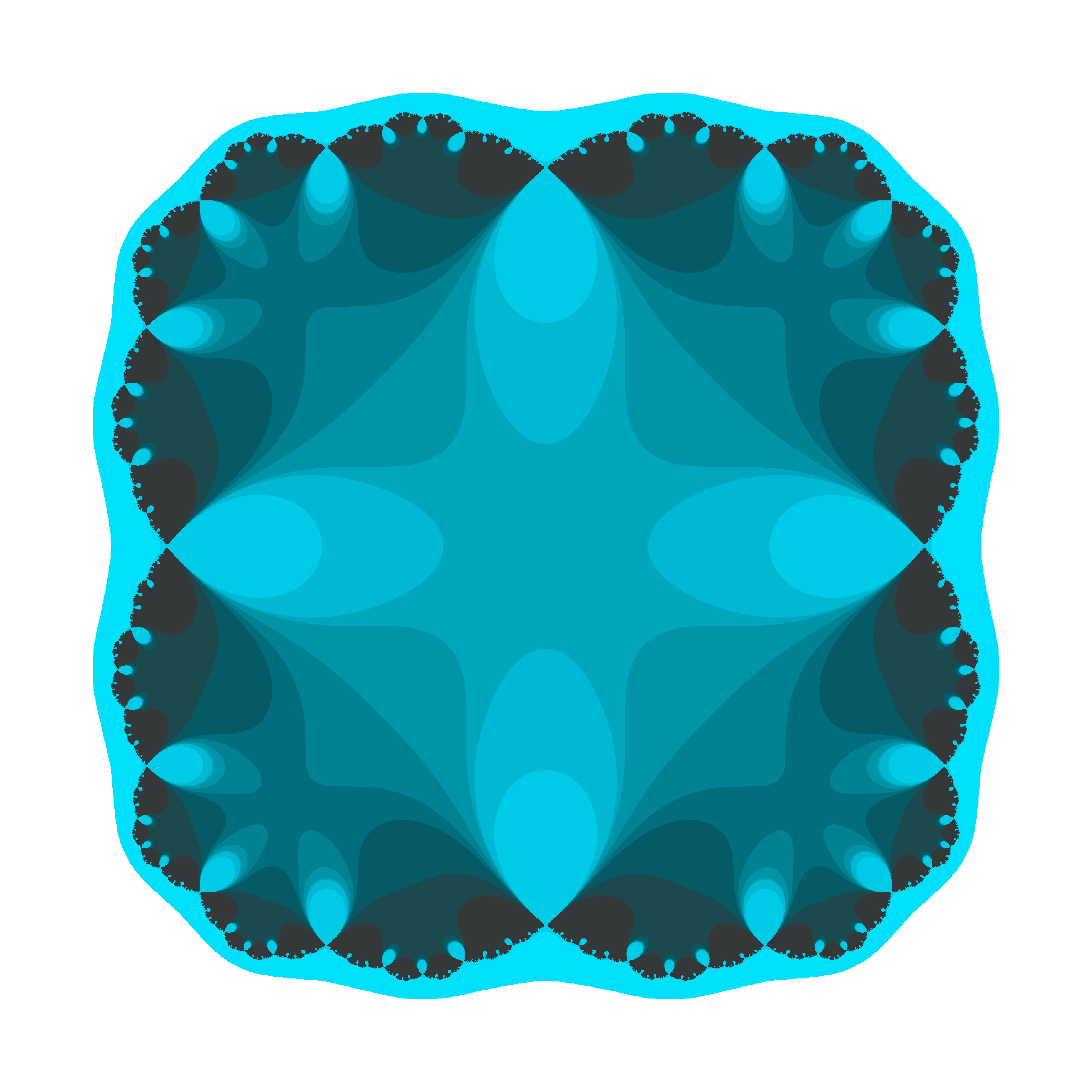There are two little Julias in the world whom I would like to dedicate this post. I wish them all the best of the world and I am sure they will discover the beauty of mathematics. These flowers are yours.

The code is available here.

# Crochet Patterns

¡Hay que ver cómo se estropean los cuerpos! (Pilar, my beloved grandmother)

My grandmother was a master of sewing. When she was young, she worked as dressmaker, and her profession became a hobby with the passage of time. I remember her doing cross-stitch, embroidering tablecloths and doing crochet. I have some of her artworks at home. She spent many hours patiently in silence, moving her knitting needles: my grandmother didn’t use to get bored. As she did with her threads, this drawing is done linking lines:You can find the code here. If you check it, you will see that the stitches of drawings are defined by a function that I called pattern, which depends on some parameters that I define randomly. This is why each time you run it, you will get a different drawing:From the technical side, I used accumulate function from purrr package, which makes loops faster and more efficient.

Drawings remind me those I created here, imitating the way that plants arrange their leaves. If you are interesting in using R to create art, check out this free DataCamp’s project.

# Tweetable Mathematical Art With R

Sin ese peso ya no hay gravedad
Sin gravedad ya no hay anzuelo
(Mira cómo vuelo, Miss Caffeina)

I love messing around with R to generate mathematical patterns. I always get surprised doing it and gives me lot of satisfaction. I also learn lot of things doing it: not only about R, but also about mathematics. It is one of my favourite hobbies. Some time ago, I published this post showing some drawings, each of them generated with less than 280 characters of code, to be shared on Twitter. This post came to appear in Hacker News, which provoked an incredible peak on visits to my blog. Some comments in the Hacker News entry are very interesting.

This Summer I delved into this concept of Tweetable Art publishing several drawings together with the R code to generate them. In this post I will show some.

Vertiginous Spiral

I came up with this image inspired by this nice pattern. It is a turtle graphic inspired pattern but instead of drawing lines I use geom_polygon to colour the resulting image in black and white:Code:

library(tidyverse)
df <- data.frame(x=0, y=0)
for (i in 2:500){
df[i,1] <- df[i-1,1]+((0.98)^i)*cos(i)
df[i,2] <- df[i-1,2]+((0.98)^i)*sin(i)
}
ggplot(df, aes(x,y)) +
geom_polygon()+
theme_void()


Slight modifications of the code can generate appealing patterns like this: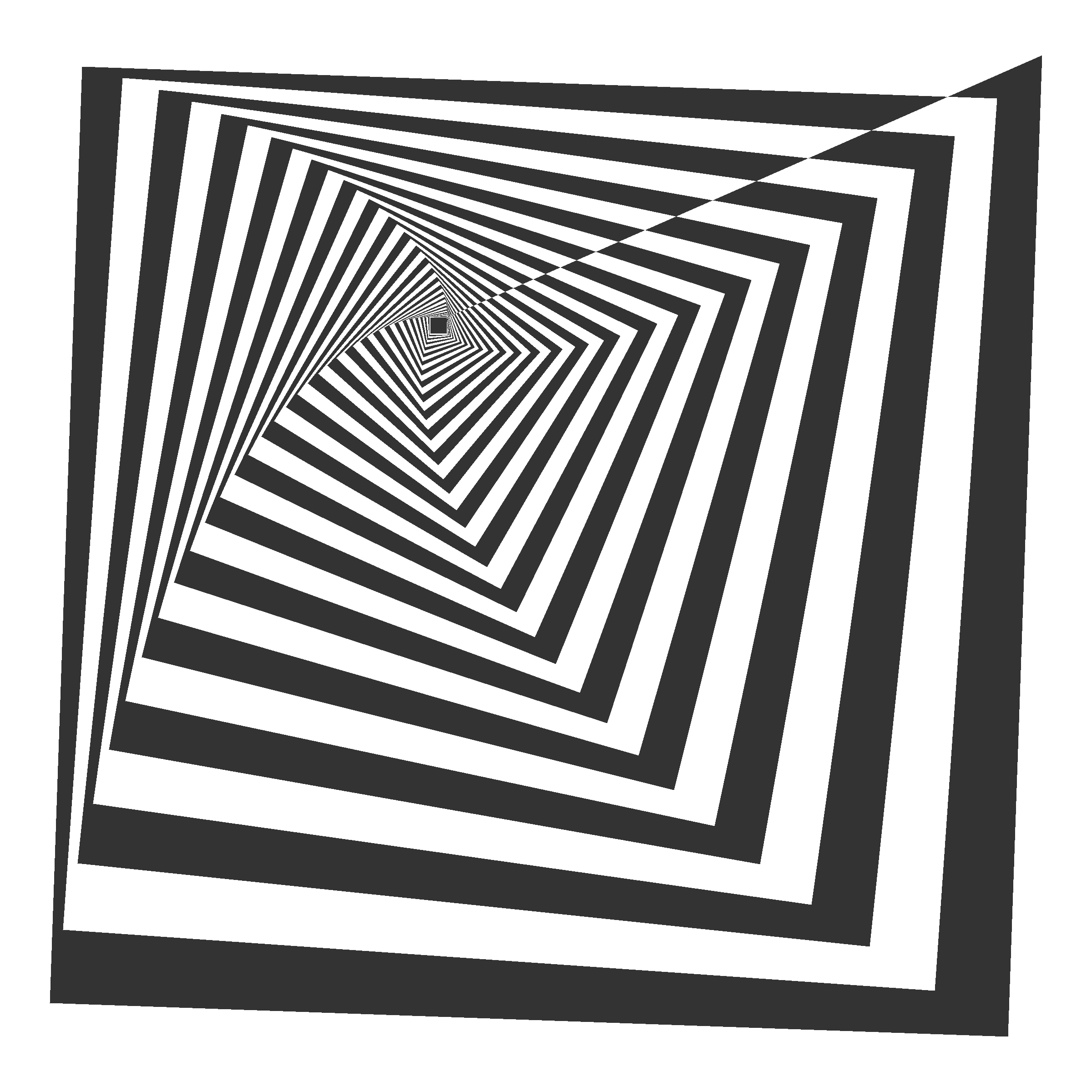Marine Creature

A combination of sines and cosines. It reminds me a jellyfish:Code:

library(tidyverse)
seq(from=-10, to=10, by = 0.05) %>%
expand.grid(x=., y=.) %>%
ggplot(aes(x=(x^2+pi*cos(y)^2), y=(y+pi*sin(x)))) +
geom_point(alpha=.1, shape=20, size=1, color="black")+
theme_void()+coord_fixed()


Summoning Cthulhu

The name is inspired in an answer from Mara Averick to this tweet. It is a modification of the marine creature in polar coordinates:Code:

library(tidyverse)
seq(-3,3,by=.01) %>%
expand.grid(x=., y=.) %>%
ggplot(aes(x=(x^3-sin(y^2)), y=(y^3-cos(x^2)))) +
geom_point(alpha=.1, shape=20, size=0, color="white")+
theme_void()+
coord_fixed()+
theme(panel.background = element_rect(fill="black"))+
coord_polar()


Naive Sunflower

Sunflowers arrange their seeds according a mathematical pattern called phyllotaxis, whic inspires this image. If you want to create your own flowers, you can do this Datacamp’s project. It’s free and will introduce you to the amazing world of ggplot2, my favourite package to create images:Code:

library(ggplot2)
a=pi*(3-sqrt(5))
n=500
ggplot(data.frame(r=sqrt(1:n),t=(1:n)*a),
aes(x=r*cos(t),y=r*sin(t)))+
geom_point(aes(x=0,y=0),
size=190,
colour="violetred")+
geom_point(aes(size=(n-r)),
shape=21,fill="gold",
colour="gray90")+
theme_void()+theme(legend.position="none")


Silk Knitting

It is inspired by this other pattern. A lot of almost transparent white points ondulating according to sines and cosines on a dark coloured background:Code:

library(tidyverse)
seq(-10, 10, by = .05) %>%
expand.grid(x=., y=.) %>%
ggplot(aes(x=(x+sin(y)), y=(y+cos(x)))) +
geom_point(alpha=.1, shape=20, size=0, color="white")+
theme_void()+
coord_fixed()+
theme(panel.background = element_rect(fill="violetred4"))


Try to modify them and generate your own patterns: it is a very funny way to learn R.

Note: in order to make them better readable, some of the pieces of code below may have more than 280 characters but removing unnecessary characters (blanks or carriage return) you can reduce them to make them tweetable.

# How Do We Draw a Line?

She dreams in colour, she dreams in red, can’t find a better man (Better Man, Pearl Jam)

Today I bring another experiment based on The Quick Draw! Data from Google, one of my most fortunate discoveries of the last times. The Quick Draw! is a web game developed by Google, that can be played on a computer, tablet or mobile phone, in which you are asked to draw something (for example, a bird). Then you have just 20 seconds to do it. You win if a machine, trained with a neural network, deduces what are you drawing. The best way to understand how it works is playing to it here. Google published data of about 50 million drawings across 345 categories, contributed by players of the game from all over the world. Datasets are in ndjson format (newline delimited JSON). In my previous post I analyzed one of these datasets, and showed a way to parse and represent the drawings in ggplot.

In this occasion I analyze the simplest drawing that Google can ask you: a line. The dataset, which is called lines.ndjson, can be found here and contains more than 143.000 lines drawn by people from about 170 countries. Most of these drawings come from The United States (45.4%), United Kingdom (7.5%), Canada (3.6%), Germany (3.5%) and Russian Federation (2.3%).

Let’s try to understand how humans draw lines. Concretely, in which direction do we draw them: horizontally? toward right o left? vertically? toward up or down? This analysis is inspired in two great articles I read recently:

There are some technical details around this experiment I would highlight:

• I parse the dataset using fromJSON function from rjson package.
• I use purrr package to apply a linear regression to the points defining the line for each drawing.
• I easily convert the summary of the linear regression into a data frame using tidy function from broom package.
• I use the slope of the regression to obtain the angle which describes the line (depending on where it is started I add pi to de arctangent of the slope)
• I represent the frequence of angles using polar coordinates dividing circle in sections of 30 degrees in the following way: 345°- 15°, 15°- 45°, 45°-75°, 75°-105°, …, 315°-345° so for example, horizontal lines from left to right will fall into 345º- 15º category.

This is how do we draw lines analysing the entire dataset, without doing any distinction by country:The fact seems clear: an average human who plays to the Quick Draw! game, draws a line horizontally from left to right with a probability of 59%. I have to admite that I expected a majority of horizontal-left-to-right lines, but not as crushingly as the plot shows. Maybe my a priori is far from the reality because I am lefty and I would draw it in another way. Remember as well that this mean human will probably come from The United States.

Are there differences by country? Yes, and they are very interesting. I removed all that countries with less then 150 drawings. Taking this into account, these are the four countries where more people draw vertical bottom-up lines:

And these are where more people draw horizontal right-left lines:

We’ve seen that on average, 59% of lines are drawn from left to right. This figure reaches more than 75% in the following countries:

And where do people draw more oblique lines? Here:

Surprisingly, a very small amount of lines are drawn toward down, which seems me quite intriguing.

Some thoughts (let me know yours):

• Humans prefer doing horizontal lines from left-to-right everywhere
• In case of drawing vertical, we clearly prefer bottom-up movement rather than the opposite; maybe the device configuration or the arrangement of the application motivates this behaviour.
• Arab and hebrew are written from right-to-left: this fact seems to have a significant influence on the way that people draw lines.

You can find the code of this experiment here.

# Exploring The Quick, Draw! Dataset With R: The Mona Lisa

All that noise, and all that sound, all those places I have found (Speed of Sound, Coldplay)

Some days ago, my friend Jorge showed me one of the coolest datasets I’ve ever seen: the Google quick draw dataset. In its Github website you can see a detailed description of the data. Briefly, it contains  around 50 million of drawings of people around the world in .ndjson format. In this experiment, I used the simplified version of drawings where strokes are simplified and resampled with a 1 pixel spacing. Drawings are also aligned to top-left corner and scaled to have a maximum value of 255. All these things make data easier to manage and to represent into a plot.

Since .ndjson files may be very large, I used LaF package to access randon lines of the file rather than reading it completely. I wrote a script to explore The Mona Lisa.ndjson file, which contains more than 120.000 drawings that the TensorFlow engine from Google recognized as being The Mona Lisa. It is quite funny to see them. Whit this script you can:

• Reproduce a random single drawing
• Create a 9×9 mosaic of random drawings
• Create an animation simulating the way the drawing was created

I use ggplot2 package to render drawings and gganimate package of David Robinson to create animations.

This is an example of a single drawing:This is an example of a 3×3 mosaic:This is an example of animation:If you want to try by yourself, you can find the code here.

Note: to work with gganimate, I downloaded the portable version and pointed to it with Sys.setenv command as explained here.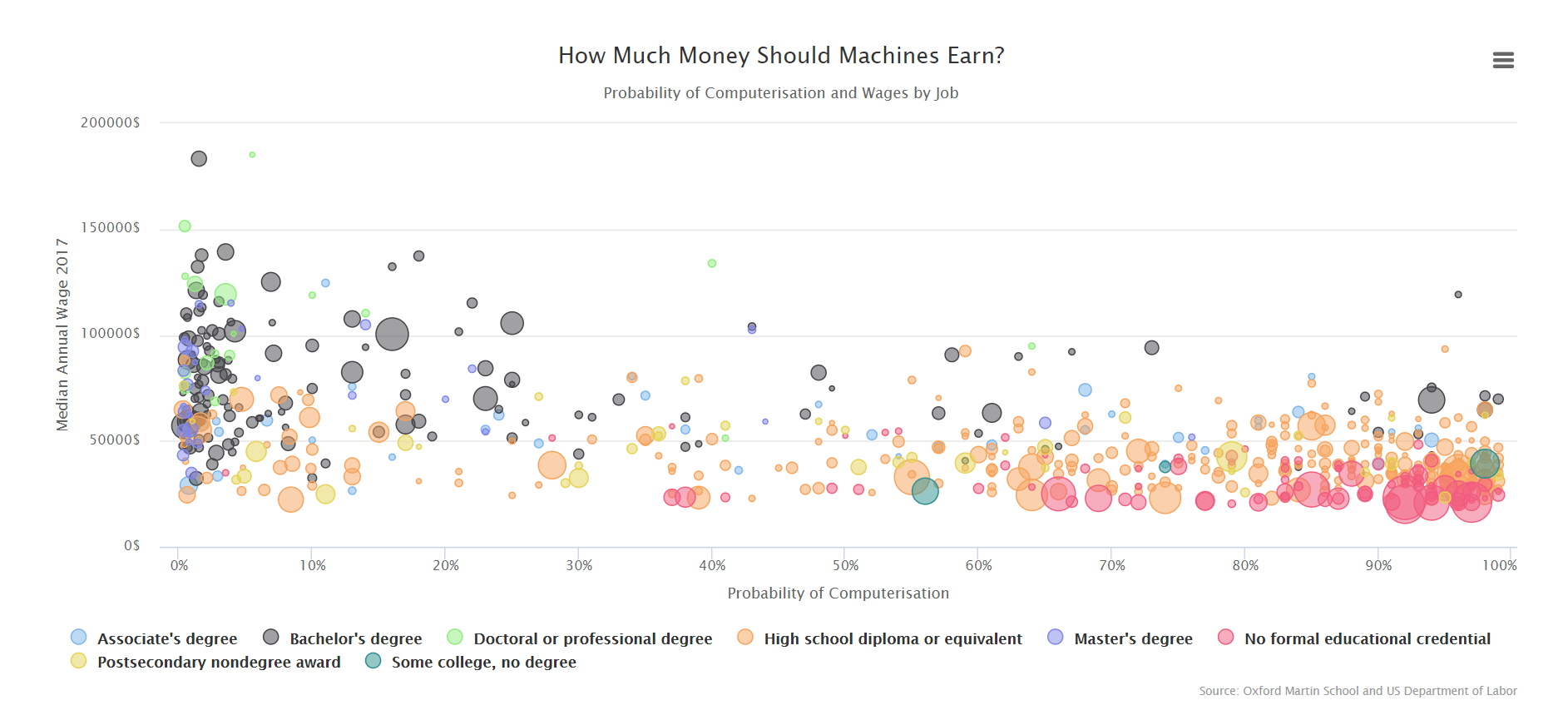# How Much Money Should Machines Earn?

Every inch of sky’s got a star
Every inch of skin’s got a scar

I think that a very good way to start with R is doing an interactive visualization of some open data because you will train many important skills of a data scientist: loading, cleaning, transforming and combinig data and performing a suitable visualization. Doing it interactive will give you an idea of the power of R as well, because you will also realise that you are able to handle indirectly other programing languages such as JavaScript.

That’s precisely what I’ve done today. I combined two interesting datasets:

• The probability of computerisation of 702 detailed occupations, obtained by Carl Benedikt Frey and Michael A. Osborne from the University of Oxford, using a Gaussian process classifier and published in this paper in 2013.
• Statistics of jobs from (employments, median annual wages and typical education needed for entry) from the US Bureau of Labor, available here.

Apart from using dplyr to manipulate data and highcharter to do the visualization, I used tabulizer package to extract the table of probabilities of computerisation from the pdf: it makes this task extremely easy.

This is the resulting plot:

If you want to examine it in depth, here you have a full size version.

These are some of my insights (its corresponding figures are obtained directly from the dataset):

• There is a moderate negative correlation between wages and probability of computerisation.
• Around 45% of US employments are threatened by machines (have a computerisation probability higher than 80%): half of them do not require formal education to entry.
• In fact, 78% of jobs which do not require formal education to entry are threatened by machines: 0% which require a master’s degree are.
• Teachers are absolutely irreplaceable (0% are threatened by machines) but they earn a 2.2% less then the average wage (unfortunately, I’m afraid this phenomenon occurs in many other countries as well).
• Don’t study for librarian or archivist: it seems a bad way to invest your time
• Mathematicians will survive to machines

The code of this experiment is available here.

# Coloring Sudokus

Someday you will find me
caught beneath the landslide
(Champagne Supernova, Oasis)

I recently read a book called Snowflake Seashell Star: Colouring Adventures in Numberland by Alex Bellos and Edmund Harris which is full of mathematical patterns to be coloured. All images are truly appealing and cause attraction to anyone who look at them, independently of their age, gender, education or political orientation. This book demonstrates how maths are an astonishing way to reach beauty.

One of my favourite patterns are tridokus, a sophisticated colored version of sudokus. Coloring a sudoku is simple: once that is solved it is enough to assign a color to each number (from 1 to 9).  If you superimpose three colored sudokus with no cells at the same position sharing the same color, and using again nine colors, the resulting image is a tridoku: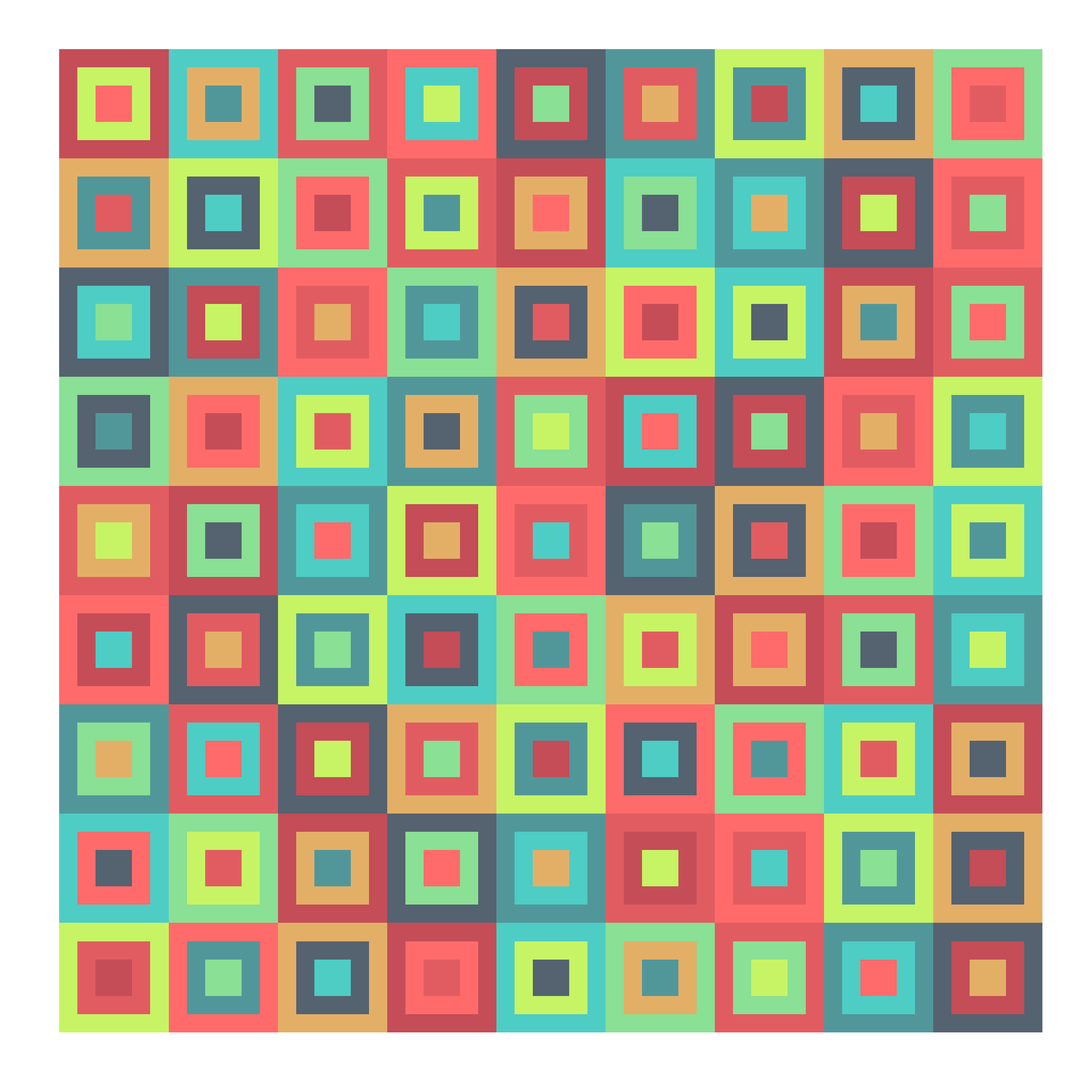There is something attractive in a tridoku due to the balance of colors but also they seem a quite messy: they are a charmingly unbalanced.  I wrote a script to generalize the concept to n-dokus. The idea is the same: superimpose n sudokus without cells sharing color and position (I call them disjoint sudokus) using just nine different colors. I did’n’t prove it, but I think the maximum amount of sudokus can be overimposed with these constrains is 9. This is a complete series from 1-doku to 9-doku (click on any image to enlarge):

I am a big fan of colourlovers package. These tridokus are colored with some of my favourite palettes from there:

Just two technical things to highlight:

• There is a package called sudoku that generates sudokus (of course!). I use it to obtain the first solved sudoku which forms the base.
• Subsequent sudokus are obtained from this one doing two operations: interchanging groups of columns first (there are three groups: columns 1 to 3, 4 to 6 and 7 to 9) and interchanging columns within each group then.

You can find the code here: do you own colored n-dokus!